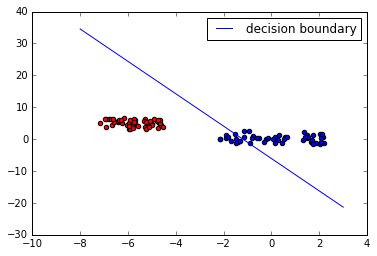## Neural Networks

### Introduction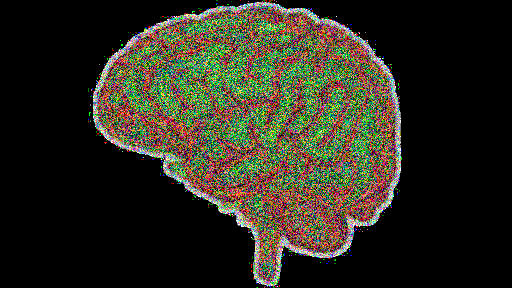When we say "Neural Networks", we mean artificial Neural Networks (ANN). The idea of ANN is based on biological neural networks like the brain.

The basic structure of a neural network is the neuron. A neuron in biology consists of three major parts: the soma (cell body), the dendrites, and the axon.

The dendrites branch of from the soma in a tree-like way and getting thinner with every branch. They receive signals (impulses) from other neurons at synapses. The axon - there is always only one - also leaves the soma and usually tend to extend for longer distances than the dentrites. The axon is used for sending the output of the neuron to other neurons or better to the synapsis of other neurons.

The following image by Quasar Jarosz, courtesy of Wikipedia, illustrates this:Even though the above image is already an abstraction for a biologist, we can further abstract it: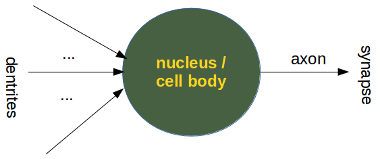A perceptron of artificial neural networks is simulating a biological neuron.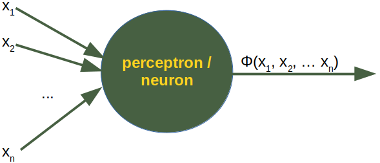It is amazingly simple, what is going on inside the body of a perceptron or neuron. The input signals get multiplied by weight values, i.e. each input has its corresponding weight. This way the input can be adjusted individually for every $x_i$. We can see all the inputs as an input vector and the corresponding weights as the weights vector.

When a signal comes in, it gets multiplied by a weight value that is assigned to this particular input. That is, if a neuron has three inputs, then it has three weights that can be adjusted individually. The weights usually get adjusted during the learn phase.
After this the modified input signals are summed up. It is also possible to add additionally a so-called bias b to this sum. The bias is a value which can also be adjusted during the learn phase.

Finally, the actual output has to be determined. For this purpose an activation or step function Φ is applied to weighted sum of the input values.The simplest form of an activation function is a binary function. If the result of the summation is greater than some threshold s, the result of $\Phi$ will be 1, otherwise 0.

$$\Phi(x) = \left\{ \begin{array}{rl} 1 &\mbox{ wx + b > s} \\ 0 &\mbox{ otherwise} \end{array} \right.$$

## A Simple Neural Network

The following image shows the general building principle of a simple artificial neural network:We will write a very simple Neural Network implementing the logical "And" and "Or" functions.

Let's start with the "And" function. It is defined for two inputs:

Input1 Input2 Output
0 0 0
0 1 0
1 0 0
1 1 1

## Line Separation

You could imagine that you have two attributes describing am eddible object like a fruit for example: "sweetness" and "sourness"

We could describe this by points in a two-dimensional space. The x axis for the sweetness and the y axis for the sourness. Imagine now that we have two fruits as points in this space, i.e. an orange at position (3.5, 1.8) and a lemon at (1.1, 3.9).

We could define dividing lines to define the points which are more lemon-like and which are more orange-like. The following program calculates and renders a bunch of lines. The red ones are completely unusable for this purpose, because they are not separating the classes. Yet, it is obvious that even the green ones are not all useful.

import numpy as np
import matplotlib.pyplot as plt

def create_distance_function(a, b, c):
""" 0 = ax + by + c """
def distance(x, y):
""" returns tuple (d, pos)
d is the distance
If pos == -1 point is below the line,
0 on the line and +1 if above the line
"""
nom = a * x + b * y + c
if nom == 0:
pos = 0
elif (nom<0 and b<0) or (nom>0 and b>0):
pos = -1
else:
pos = 1
return (np.absolute(nom) / np.sqrt( a ** 2 + b ** 2), pos)
return distance

points = [ (3.5, 1.8), (1.1, 3.9) ]

fig, ax = plt.subplots()
ax.set_xlabel("sweetness")
ax.set_ylabel("sourness")
ax.set_xlim([-1, 6])
ax.set_ylim([-1, 8])
X = np.arange(-0.5, 5, 0.1)

colors = ["r", ""] # for the samples

size = 10
for (index, (x, y)) in enumerate(points):
if index== 0:
ax.plot(x, y, "o",
color="darkorange",
markersize=size)
else:
ax.plot(x, y, "oy",
markersize=size)

step = 0.05
for x in np.arange(0, 1+step, step):
slope = np.tan(np.arccos(x))
dist4line1 = create_distance_function(slope, -1, 0)
#print("x: ", x, "slope: ", slope)
Y = slope * X

results = []
for point in points:
results.append(dist4line1(*point))
#print(slope, results)
if (results != results):
ax.plot(X, Y, "g-")
else:
ax.plot(X, Y, "r-")

plt.show()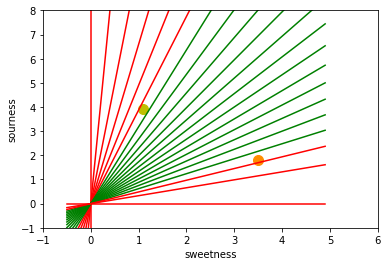In the following program, we train a neural network to classify two clusters in a 2-dimensional space. We show this in the following diagram with the two classes class1 and class2. We will create those points randomly with the help of a line, the points of class2 will be above the line and the points of class1 will be below the line.

import numpy as np

class Perceptron:

def __init__(self, input_length, weights=None):
if weights is None:
self.weights = np.ones(input_length) * 0.5
else:
self.weights = weights

@staticmethod
def unit_step_function(x):
if x > 0.5:
return 1
return 0

def __call__(self, in_data):
weighted_input = self.weights * in_data
weighted_sum = weighted_input.sum()
return Perceptron.unit_step_function(weighted_sum)

p = Perceptron(2, np.array([0.5, 0.5]))

data_in = np.empty((2,))
for in1 in range(2):
for in2 in range(2):
data_in = (in1, in2)
data_out = p(data_in)
print(data_in, data_out)

(0, 0) 0
(0, 1) 0
(1, 0) 0
(1, 1) 1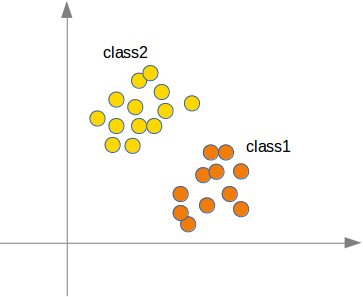We will see that the neural network will find a line that separates the two classes. This line should not be mistaken for the line, which we used to create the points.

This line is called a decision boundary.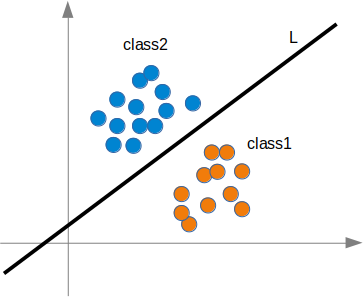import numpy as np
from collections import Counter

class Perceptron:

def __init__(self, input_length, weights=None):
if weights==None:
self.weights = np.random.random((input_length)) * 2 - 1
self.learning_rate = 0.1

@staticmethod
def unit_step_function(x):
if x < 0:
return 0
return 1

def __call__(self, in_data):
weighted_input = self.weights * in_data
weighted_sum = weighted_input.sum()
return Perceptron.unit_step_function(weighted_sum)

target_result,
calculated_result,
in_data):
error = target_result - calculated_result
for i in range(len(in_data)):
correction = error * in_data[i] *self.learning_rate
self.weights[i] += correction

def above_line(point, line_func):
x, y = point
if y > line_func(x):
return 1
else:
return 0

points = np.random.randint(1, 100, (100, 2))
p = Perceptron(2)

def lin1(x):
return  x + 4

for point in points:
p(point),
point)

evaluation = Counter()
for point in points:
if p(point) == above_line(point, lin1):
evaluation["correct"] += 1
else:
evaluation["wrong"] += 1

print(evaluation.most_common())

[('correct', 100)]


The decision boundary of our previous network can be calculated by looking at the following condition

$$x_1 w_1 + x_2w_2 = 0$$

We can change the equation into

$$x_2 = -\frac{w_1}{w_2}x_1$$

When we look at the general form of a straight line $y = mx + b$, we can easily see that our equation corresponds to the definition of a line and the slope (aka gradient) $m$ is $-\frac{w_1}{w_2}$ and $b$ is equal to 0.

### Single Layer with Bias

As the constant term $b$ determines the point at which a line crosses the y-axis, i.e. the y-intercept, we can see that our network can only calculate lines which pass through the origin, i.e. the point (0, 0). We will need a bias to get other lines as well, i.e. lines which don't go through the origin. A neural network with bias nodes can look like this: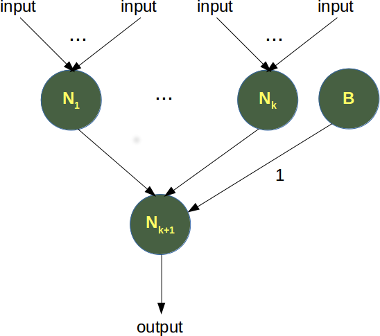Now, the linear equation for a perceptron contains a bias:

$$b + \sum_{i=1}^{n} x_i \cdot w_i = 0$$

We add now some code to print the points and the dividing line according to the previous equation:

# the following line is only needed,
# if you use "ipython notebook":
%matplotlib inline

from matplotlib import pyplot as plt

cls = [[], []]
for point in points:
cls[above_line(point, lin1)].append(tuple(point))

colours = ("r", "b")
for i in range(2):
X, Y = zip(*cls[i])
plt.scatter(X, Y, c=colours[i])

X = np.arange(-3, 120)

m = -p.weights / p.weights
print(m)
plt.plot(X, m*X, label="ANN line")
plt.plot(X, lin1(X), label="line1")
plt.legend()
plt.show()

1.11082111934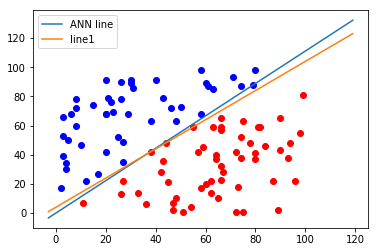We create a new dataset for our next experiments:

from matplotlib import pyplot as plt

class1 = [(3, 4), (4.2, 5.3), (4, 3), (6, 5), (4, 6), (3.7, 5.8),
(3.2, 4.6), (5.2, 5.9), (5, 4), (7, 4), (3, 7), (4.3, 4.3) ]
class2 = [(-3, -4), (-2, -3.5), (-1, -6), (-3, -4.3), (-4, -5.6),
(-3.2, -4.8), (-2.3, -4.3), (-2.7, -2.6), (-1.5, -3.6),
(-3.6, -5.6), (-4.5, -4.6), (-3.7, -5.8) ]

X, Y = zip(*class1)
plt.scatter(X, Y, c="r")

X, Y = zip(*class2)
plt.scatter(X, Y, c="b")
plt.show()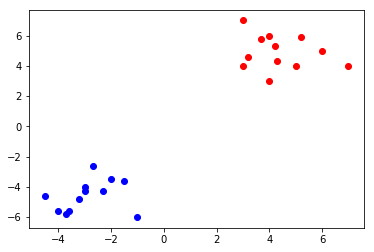from itertools import chain

p = Perceptron(2)

def lin1(x):
return  x + 4

for point in class1:
p(point),
point)

for point in class2:
p(point),
point)

evaluation = Counter()
for point in chain(class1, class2):
if p(point) == 1:
evaluation["correct"] += 1
else:
evaluation["wrong"] += 1

testpoints = [(3.9, 6.9), (-2.9, -5.9)]
for point in testpoints:
print(p(point))

print(evaluation.most_common())

1
0
[('correct', 12), ('wrong', 12)]

from matplotlib import pyplot as plt

X, Y = zip(*class1)
plt.scatter(X, Y, c="r")

X, Y = zip(*class2)
plt.scatter(X, Y, c="b")

x = np.arange(-7, 10)
y = 5*x + 10

m = -p.weights / p.weights
plt.plot(x, m*x)
plt.show()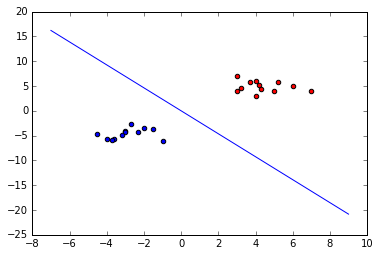from matplotlib import pyplot as plt

class1 = [(3, 4, 3), (4.2, 5.3, 2.5), (4, 3, 3.8),
(6, 5, 2.7), (4, 6, 2.9), (3.7, 5.8, 4.2),
(3.2, 4.6, 1.9), (5.2, 5.9, 2.7), (5, 4, 3.5),
(7, 4, 2.7), (3, 7, 3.1), (4.3, 4.3, 3.8) ]
class2 = [(-3, -4, 7.6), (-2, -3.5, 6.9), (-1, -6, 8.6),
(-3, -4.3, 7.4), (-4, -5.6, 7.9), (-3.2, -4.8, 5.3),
(-2.3, -4.3, 8.1), (-2.7, -2.6, 7.3), (-1.5, -3.6, 7.8),
(-3.6, -5.6, 6.8), (-4.5, -4.6, 8.3), (-3.7, -5.8, 8.7) ]

X, Y, Z = zip(*class1)
plt.scatter(X, Y, Z, c="r")

X, Y, Z = zip(*class2)
plt.scatter(X, Y, Z, c="b")
plt.show()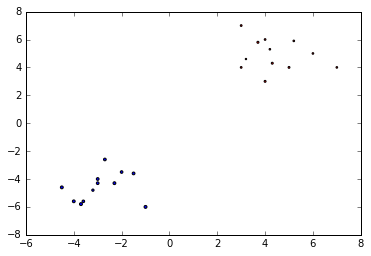### Linearly Separable and Inseparable Neural Networks

If two data clusters (classes) can be separated by a decision boundary in the form of a linear equation

$$\sum_{i=1}^{n} x_i \cdot w_i = 0$$

they are called linearly separable.

Otherwise, i.e. if such a decision boundary does not exist, the two classes are called linearly inseparable. In this case, we cannot use a simple neural network.

In the following section, we will introduce the XOR problem for neural networks. It is the simplest example of a non linearly separable neural network. I can be solved with an additional layer of neurons, which is called a hidden layer.

### The XOR Problem for Neural Networks

The XOR (exclusive or) function is defined by the following truth table:

Input1 Input2 XOR Output
0 0 0
0 1 1
1 0 1
1 1 0

This problem can't be solved with a simple neural network. We need to introduce a new type of neural networks, a network with so-called hidden layers. A hidden layer allows the network to reorganize or rearrange the input data.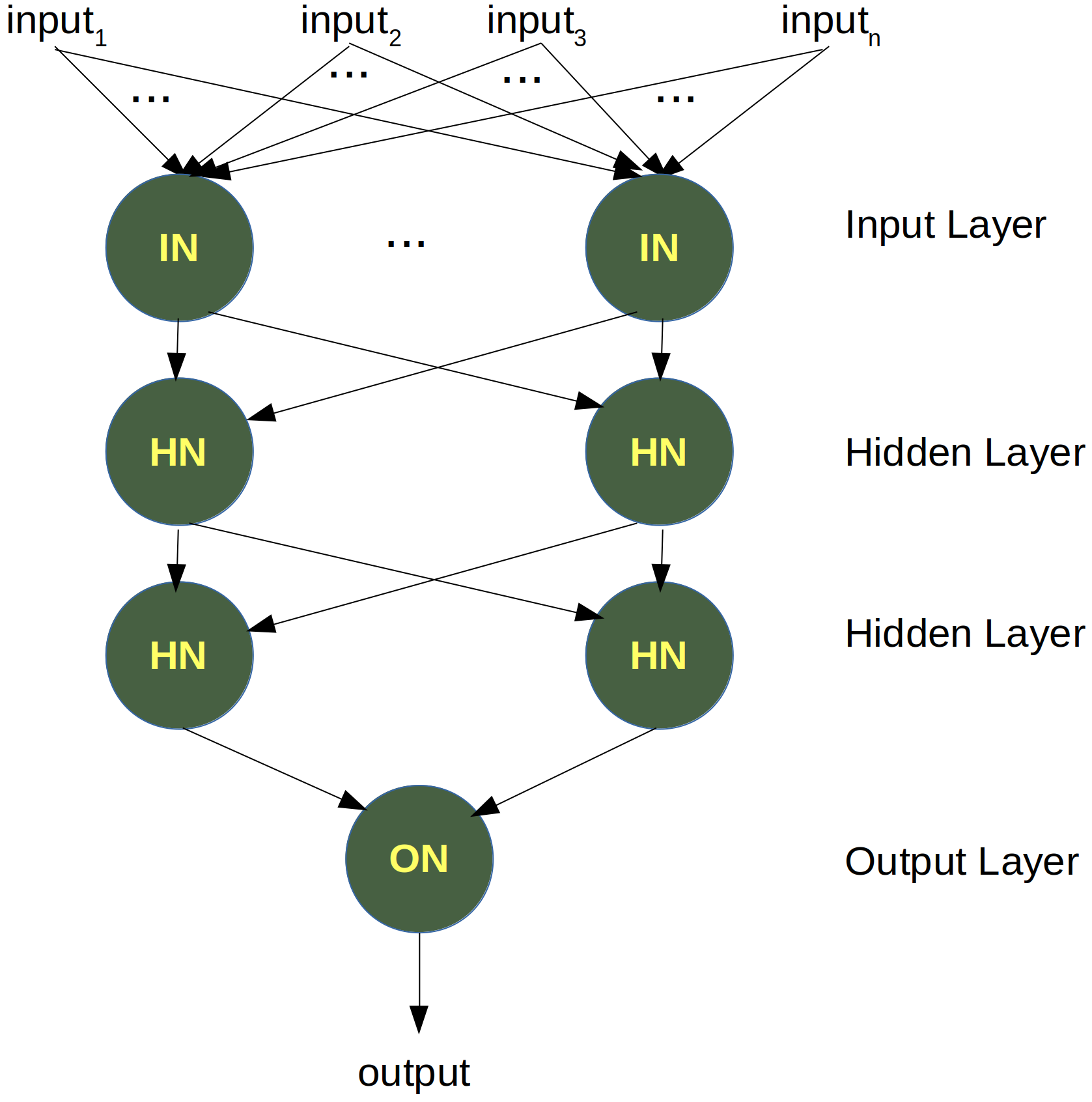We will need only one hidden layer with two neurons. One works like an AND gate and the other one like an OR gate. The output will "fire", when the OR gate fires and the AND gate doesn't.ANN with hidden layers:

The task is to find a line which separates the orange points from the blue points. But they can be separated by two lines, e.g. L1 and L2 in the following diagram: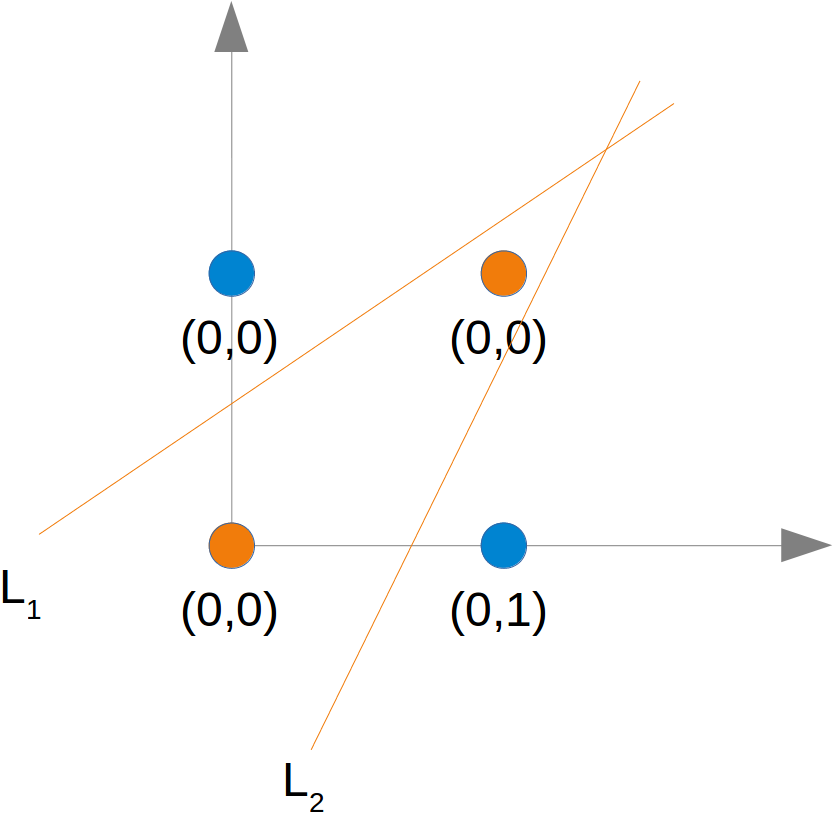To solve this problem, we need a network of the following kind, i.e with a hidden layer N1 and N2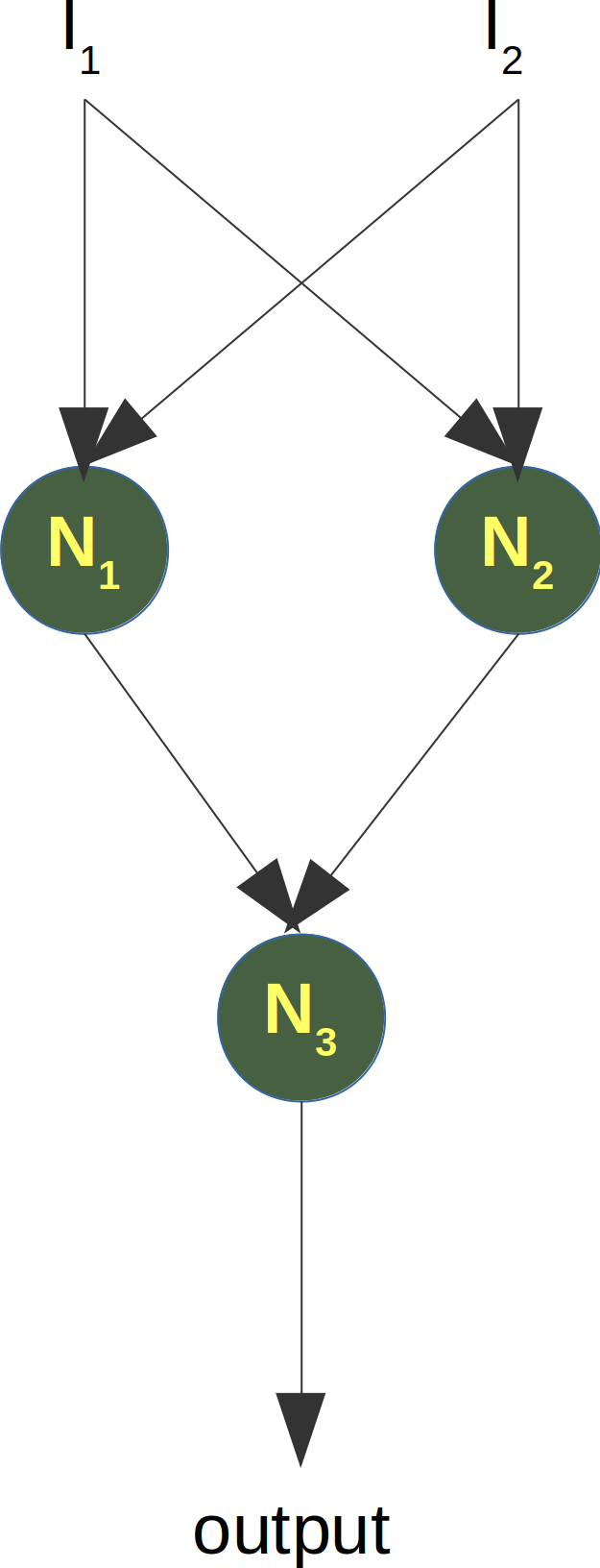The neuron N1 will determine one line, e.g. L1 and the neuron N2 will determine the other line L2. N3 will finally solve our problem: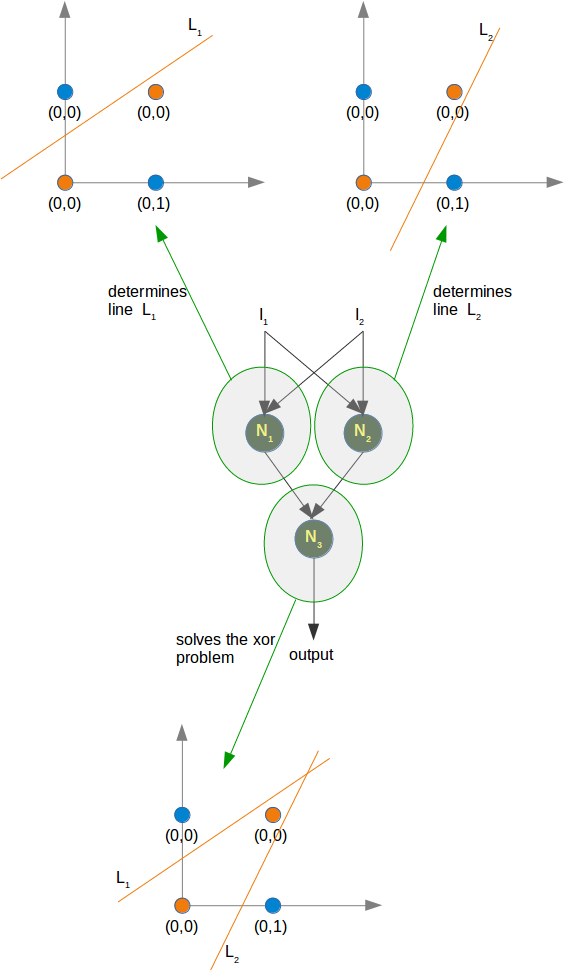### Neural Network with Bias Values

We will come back now to our initial example with the random points above and below a line. We will rewrite the code using a bias value.

First we will create two classes with random points, which are not separable by a line crossing the origin.

We will add a bias b to our neural network. This leads us to the following condition

$$x_1 w_1 + x_2w_2 + b w_3= 0$$

We can change the equation into

$$x_2 = -\frac{w_1}{w_2}x_1 -\frac{w_3}{w_2}b$$
import numpy as np
from matplotlib import pyplot as plt

npoints = 50
X, Y = [], []
# class 0
X.append(np.random.uniform(low=-2.5, high=2.3, size=(npoints,)) )
Y.append(np.random.uniform(low=-1.7, high=2.8, size=(npoints,)))

# class 1
X.append(np.random.uniform(low=-7.2, high=-4.4, size=(npoints,)) )
Y.append(np.random.uniform(low=3, high=6.5, size=(npoints,)))

learnset = []
for i in range(2):
# adding points of class i to learnset
points = zip(X[i], Y[i])
for p in points:
learnset.append((p, i))

colours = ["b", "r"]
for i in range(2):
plt.scatter(X[i], Y[i], c=colours[i])


import numpy as np
from collections import Counter

class Perceptron:

def __init__(self, input_length, weights=None):
if weights==None:
self.weights = np.random.random((input_length)) * 2 - 1
self.learning_rate = 0.1

@staticmethod
def unit_step_function(x):
if x < 0:
return 0
return 1

def __call__(self, in_data):
weighted_input = self.weights * in_data
weighted_sum = weighted_input.sum()
return Perceptron.unit_step_function(weighted_sum)

target_result,
calculated_result,
in_data):
error = target_result - calculated_result
for i in range(len(in_data)):
correction = error * in_data[i] *self.learning_rate
self.weights[i] += correction

p = Perceptron(2)

for point, label in learnset:
p(point),
point)

evaluation = Counter()
for point, label in learnset:
if p(point) == label:
evaluation["correct"] += 1
else:
evaluation["wrong"] += 1

print(evaluation.most_common())

colours = ["b", "r"]
for i in range(2):
plt.scatter(X[i], Y[i], c=colours[i])

XR = np.arange(-8, 4)
m = -p.weights / p.weights
print(m)
plt.plot(XR, m*XR, label="decision boundary")
plt.legend()
plt.show()

[('correct', 77), ('wrong', 23)]
3.10186712936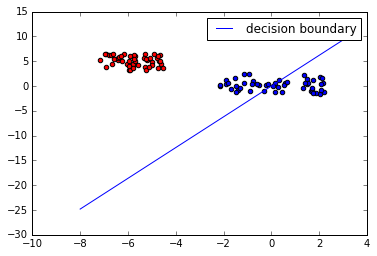It is not possible to find a solution with one neuron and without a bias node. The reason is that the class of the blue data points spread around the origin. Without bias nodes we get only lines going through the origin as we have mentioned earlier. It is easy to see that no line going through the origin can separate the blue from the red data.

The following class uses bias nodes and solves this problem:

import numpy as np
from collections import Counter

class Perceptron:

def __init__(self, input_length, weights=None):
if weights==None:
# input_length + 1 because bias needs a weight as well
self.weights = np.random.random((input_length + 1)) * 2 - 1
self.learning_rate = 0.05
self.bias = 1

@staticmethod
def sigmoid_function(x):
res = 1 / (1 + np.power(np.e, -x))
return 0 if res < 0.5 else 1

def __call__(self, in_data):
weighted_input = self.weights[:-1] * in_data
weighted_sum = weighted_input.sum() + self.bias *self.weights[-1]
return Perceptron.sigmoid_function(weighted_sum)

target_result,
calculated_result,
in_data):
error = target_result - calculated_result
for i in range(len(in_data)):
correction = error * in_data[i]  *self.learning_rate
#print("weights: ", self.weights)
#print(target_result, calculated_result, in_data, error, correction)
self.weights[i] += correction
# correct the bias:
correction = error * self.bias * self.learning_rate
self.weights[-1] += correction

p = Perceptron(2)

for point, label in learnset:
p(point),
point)

evaluation = Counter()
for point, label in learnset:
if p(point) == label:
evaluation["correct"] += 1
else:
evaluation["wrong"] += 1

print(evaluation.most_common())

colours = ["b", "r"]
for i in range(2):
plt.scatter(X[i], Y[i], c=colours[i])

XR = np.arange(-8, 4)
m = -p.weights / p.weights

b = -p.weights[-1]/p.weights
print(m, b)
plt.plot(XR, m*XR + b, label="decision boundary")
plt.legend()
plt.show()

[('correct', 90), ('wrong', 10)]
-5.07932788718 -6.08697420041